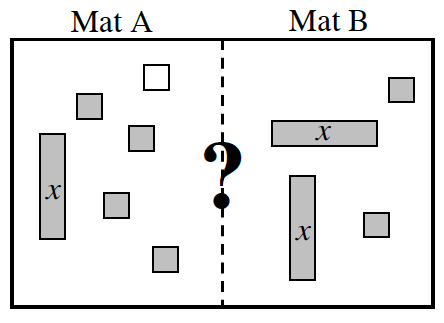### Home > CC2 > Chapter Ch6 > Lesson 6.2.4 > Problem6-102

6-102.

Write an expression for each mat below.

1. Simplify each mat to determine which expression is greater, if possible.

Cancel out any zero pairs that you find.

How many of each tile is left from each side? Use this information to write an expression for each mat.

Mat A has the expression $x+3$ since there is one $x$-tile and $3$ unit tiles. Mat B has the expression $2x+2$. Now remove any matched sets from both sides.

We are left with:
Mat A equals $1$
Mat B equals $x$
Since we don't know the value of $x$, we do not know which Mat is greater.

2. If $x=3$, would your answer to part (a) change? Explain.

Which Mat is greater when you substitute $3$ for $x$?

3. If $x=−2$, would your answer change? Explain.

Which Mat is greater when you substitute $−2$ for $x$?Explore the problem with the eTool below.
Click the link at right for the full version of the eTool: 6-102 HW eTool (CPM)